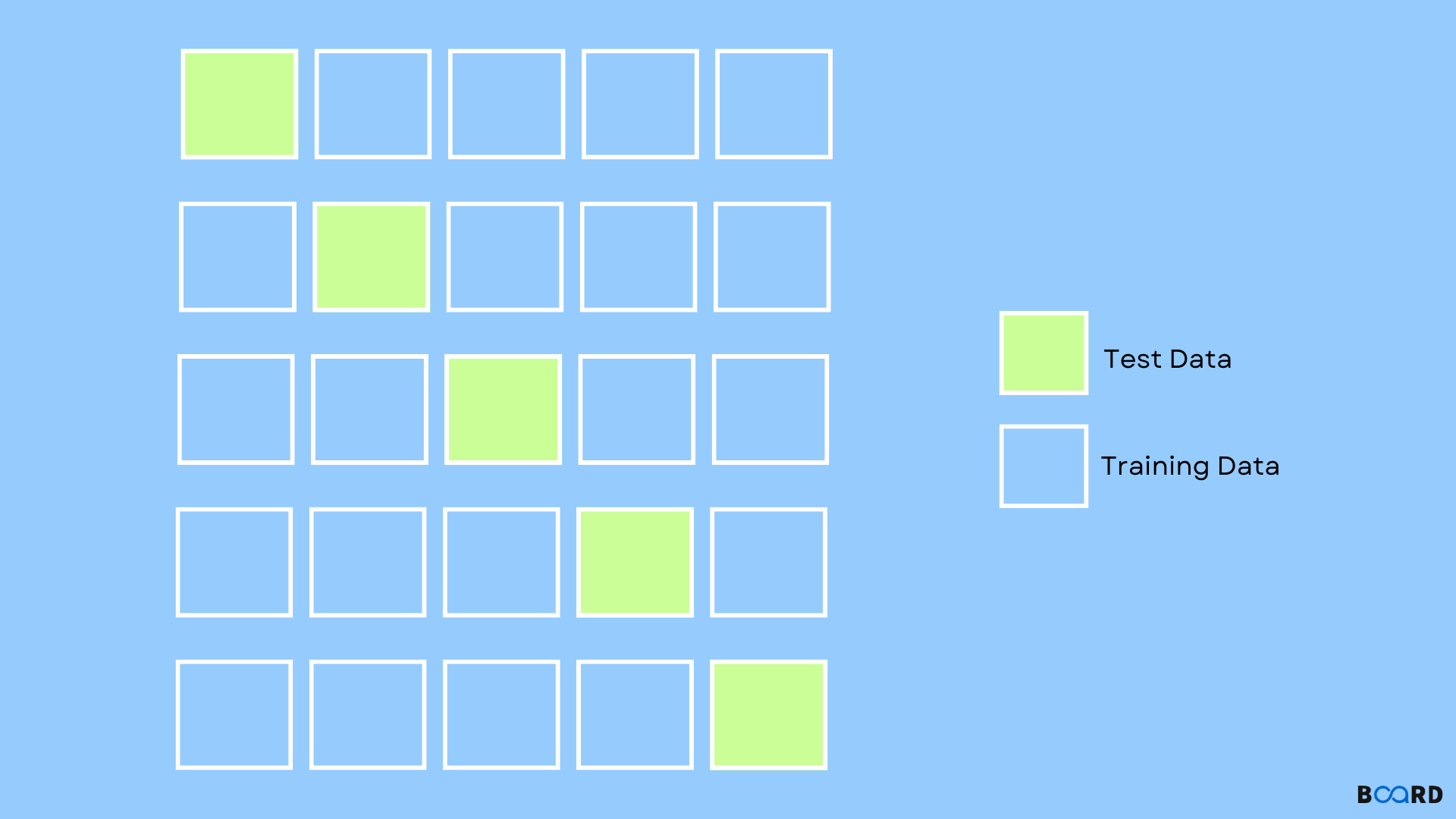# Cross Validation In Machine Learning## Overview

In M.L. (Machine Learning), cross-validation is a technique used for the validation of the efficiency of a model, by training it on a subset of the input training data, and then testing the model upon a previously unknown subset of the same input training data. Think of it as a technique for verifying the capability of a statistical model to generalize to an independent dataset.

## Scope

• What is cross-validation in ML?
• Methods of cross-validation ML:
1. Validation.
2. The method of L.O.O.C.V. (Leave One Out Cross Validation).
3. The K-Fold method.

## What is cross-validation ML?

There always exists the requirement of testing the stability of an ML model, meaning that only on basis of the training dataset, one cannot fit one’s ML model upon the training dataset. For doing so, it is required to reserve a certain portion of a dataset that is not a part of the training dataset. This is followed by testing of the model on the reserved sample dataset, before deployment. This sequence of steps is what cross-validation in ML is!

The following are the 3 steps involved in ML cross-validation:

1. Reserve a part of a sample dataset.
2. Use the remaining sample dataset for training the model.
3. Using the previously reserved sample dataset part, test the ML model.

## Methods Of Cross-Validation

### Validation

In validation, we train upon half of a given dataset. The other half is used for testing purposes. A major drawback of validation is that since we train upon one-half of the dataset, there’s a possibility that the remaining dataset contains some relevant information that was left out of the training of the model.

### LOOCV

In the method of L.O.O.C.V. (Leave One Out Cross Validation), the model is trained using the entire dataset, while leaving out only one data point of the dataset, and then iterating for each data point. One prominent benefit of this method is that all the data points are used, thus it is low bias.

A big drawback of LOOCV is that it leads to higher variation in the testing model since testing is done only against a single data point. If that data point is an outlier, it could cause higher variation. Another disadvantage is that LOOCV consumes a great amount of execution time because the number of iterations done is the same as the number of data points.

### K-Fold Cross Validation

In the K-Fold method, the dataset is divided into ‘k’ subsets, called folds. Using all, but one fold, training is done. The fold left out is used in the evaluation of the model once it is trained. This method performs k iterations k times, in each of which, a different subset is reserved for testing.